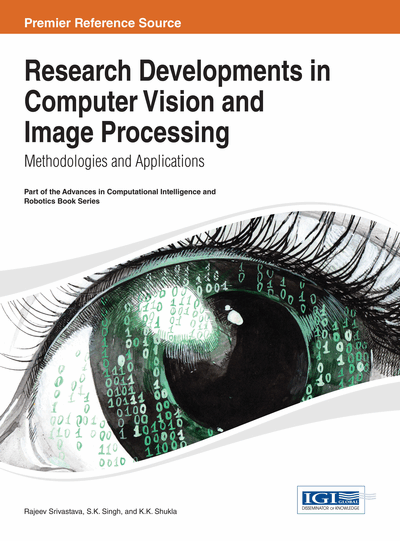# Image Representation Using a Sparsely Sampled Codebook for Super-Resolution

Hwa-Young Kim, Rae-Hong Park, Ji-Eun Lee
DOI: 10.4018/978-1-4666-4558-5.ch001
OnDemand:
(Individual Chapters)
Available
\$29.50
No Current Special Offers

## Abstract

In this chapter, the authors propose a Super-Resolution (SR) method using a vector quantization codebook and filter dictionary. In the process of SR, we use the idea of compressive sensing to represent a sparsely sampled signal under the assumption that a combination of a small number of codewords can represent an image patch. A low-resolution image is obtained from an original high-resolution image, degraded by blurring and down-sampling. The authors propose a resolution enhancement using an alternative l1 norm minimization to overcome the convexity of l0 norm and the sparsity of l1 norm at the same time, where an iterative reweighted l1 norm minimization is used for optimization. After the reconstruction stage, because the optimization is implemented on image patch basis, an additional deblurring or denoising step is used to globally enhance the image quality. Experiment results show that the proposed SR method provides highly efficient results.
Chapter Preview
Top

## Introduction

Super-resolution (SR) has been studied for several decades and a large number of SR algorithms have been proposed (Wang & Wang, 2009; Sroubek et al., 2011; Ma et al., 2012; Blunt, 2011). SR is an inverse problem, in which an original image is recovered using a single or multiple Low-Resolution (LR) images. Reconstruction is based on an image generation model that relates a High-Resolution (HR) image to a single or multiple LR images. Most conventional approaches to generating an SR image using multiple LR images require several LR images of the same scene, typically registered with sub-pixel accuracy.

There are two main processes on SR using multiple images: registration and reconstruction. Registration estimates motion of LR images with respect to the reference image. After motion estimation, the reconstruction step such as optimization, adaptive filtering, and example based method gives the HR image.

Most of SR methods use optimization to find the best HR image from initial images because the given LR images cannot offer much information for reconstruction of the exact HR image. Many conventional optimization methods define a cost function for reconstruction from distorted LR images. In l2 norm minimization with Gaussian distribution error assumption, the reconstructed HR image is an average of the contributions from all LR images, in which the Gaussian distribution error assumption is applied to LR input images with global motion. l1 norm with Laplacian distribution error assumption is good for local motion because of robustness against outliers. However, it uses the median over the measured data, so failure occurs in occlusion cases. To consider both distortion assumptions in a video SR method, Omer and Tanaka (2008) proposed a general cost function that consists of weighted l1- and l2-norms considering the SR error model, where weights are generated from the registration error with a penalty to inaccurately registered parts.

Qiao et al. (2005) proposed a SR method using Bayesian maximum a posteriori (MAP) to reconstruct a HR image and Vector Quantization (VQ) to implement blur identification. Example based SR methods, which store patch vectors from a number of training images, search for the most similar patch vector from LR image patch vectors. These methods store feature vectors (gradients) of the patch vector or LR and HR patch vector pairs (Chang et al., 2004; Yang et al., 2008).

Compressive Sensing (CS) is a new approach to recovery of a sparsely sampled signal by reducing the number of samples from bases or dictionary. CS assumes the signal could be described by combination of bases. Thus, though the recovery process is an ill-posed problem, optimization using norm minimization can give good result (Yang et al., 2009, 2012). In image processing fields, the discrete Fourier transform, Discrete Wavelet Transform (DWT), and curvelet can be used for construction of bases. To recover a sparsely sampled signal, l0 and l1 norm minimizations are presented (Candes & Romberg, 2004, 2005). Also projection on convex set (Tang et al., 2011) and efficient projection (Nhat & Vo, 2008) are used for optimization.

Recently, SR methods using CS are proposed. Since an image is two-dimensional (2-D), it is difficult to describe an image as a sparsely sampled signal on the spatial domain. Instead of the spatial domain, Duarte et al. (2008) applied a hidden Markov tree model to the DWT to use spatial data of an image and to estimate DWT coefficients. Yang et al. (2008) and Mairal et al. (2008) constructed a dictionary so that a linear combination of dictionary represents an image, where CS searches for the combination of dictionary elements.

In this chapter, it is assumed that an image can be represented using a small number of codewords that are selected from a VQ codebook. Thus, an iterative reweighted l1 (IRWL1) norm minimization is used to find a suitable combination of code-words.

## Complete Chapter List

Search this Book:
Reset